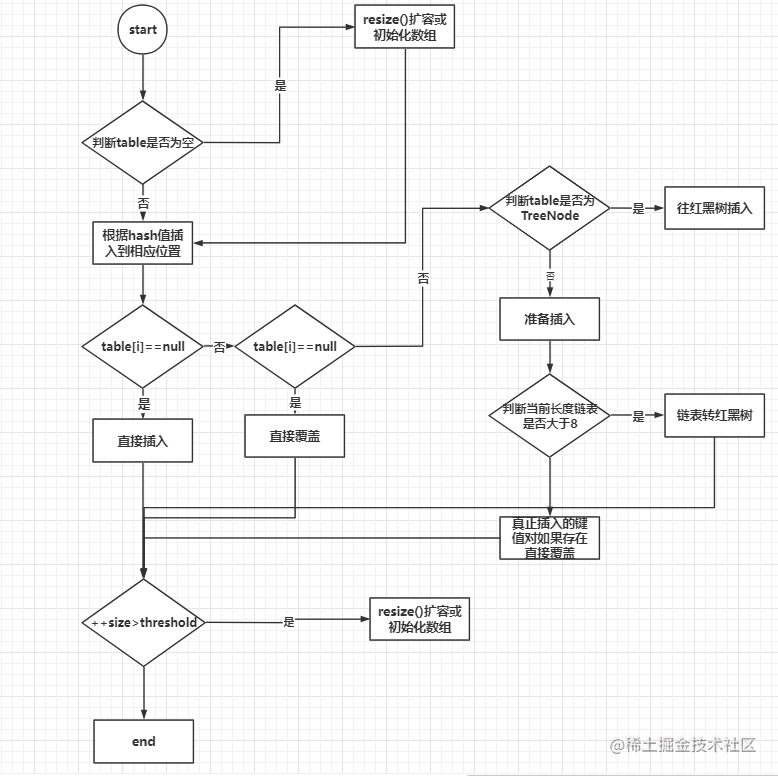# HashMap总结

#### JDK1.7中的HashMap

##### 重要成员属性
``````// 默认初始容量 初始容量必须是2的幂
static final int DEFAULT_INITIAL_CAPACITY = 1 << 4; // aka 16
// 最大hash表容量
static final int MAXIMUM_CAPACITY = 1 << 30;
// 默认加载因子（取了在时间和空间上一个比较不错的均衡，不一定是0.75，不同的hashmap实现也存在不同的值）
static final float DEFAULT_LOAD_FACTOR = 0.75f;
// 空hash表
static final Entry<?,?>[] EMPTY_TABLE = {};
// hash表，需要扩容长度必须始终是 2 的幂。
transient Entry<K,V>[] table = (Entry<K,V>[]) EMPTY_TABLE;

##### 构造函数
``````Map<String, String> map = new HashMap<String, String>();

Map<String, String> map = new HashMap<String, String>(11);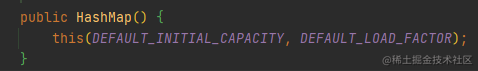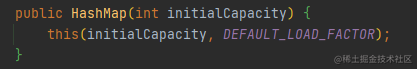##### put()方法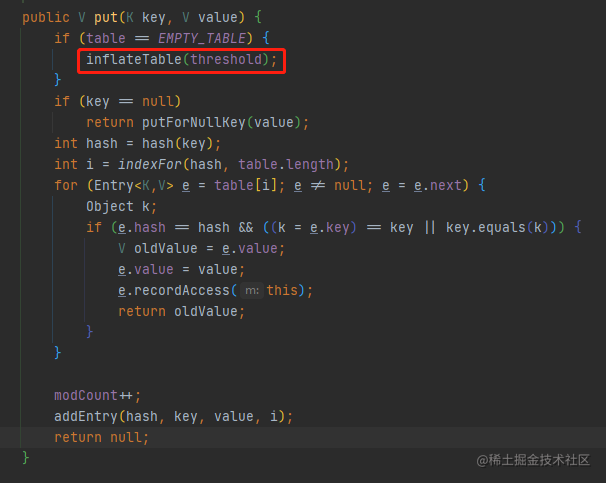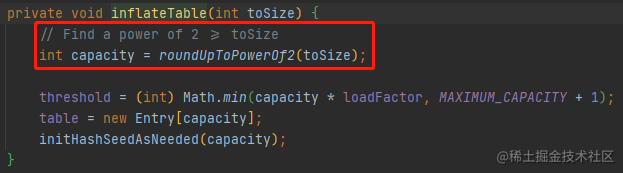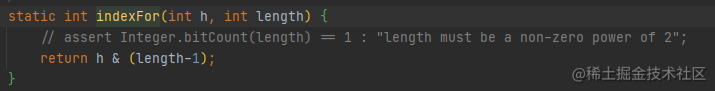``````h      = 0001 0101 0111 0010 1111
length = 0000 0000 0000 0001 0000 (16不减1) 结果只有两种0，16
length = 0000 0000 0000 0000 1111 (16减1=15) 结果有16种，更加散列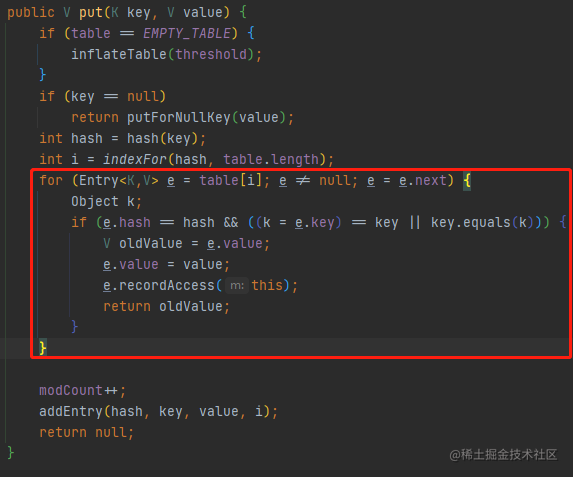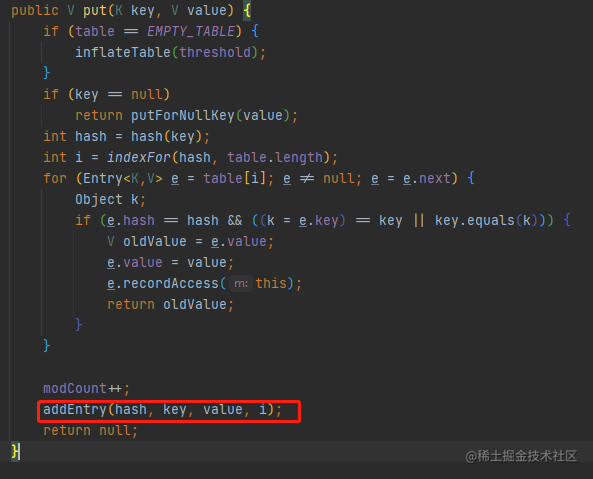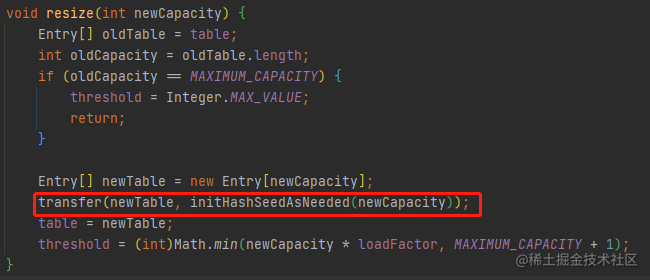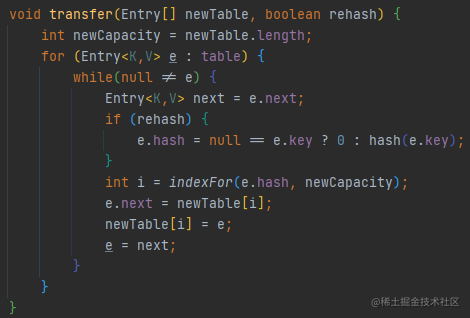##### 单线程扩容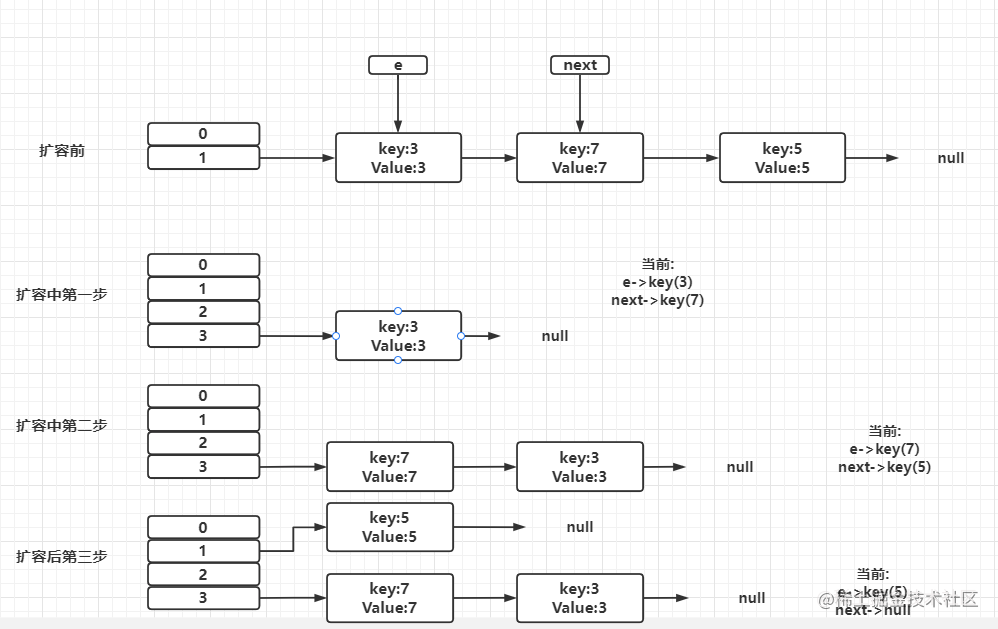##### 多线程扩容
``````while(null != e) {
Entry<K,V> next = e.next;//第一行，线程1执行到此被调度挂起
int i = indexFor(e.hash, newCapacity);//第二行
e.next = newTable[i];//第三行
newTable[i] = e;//第四行
e = next;//第五行
}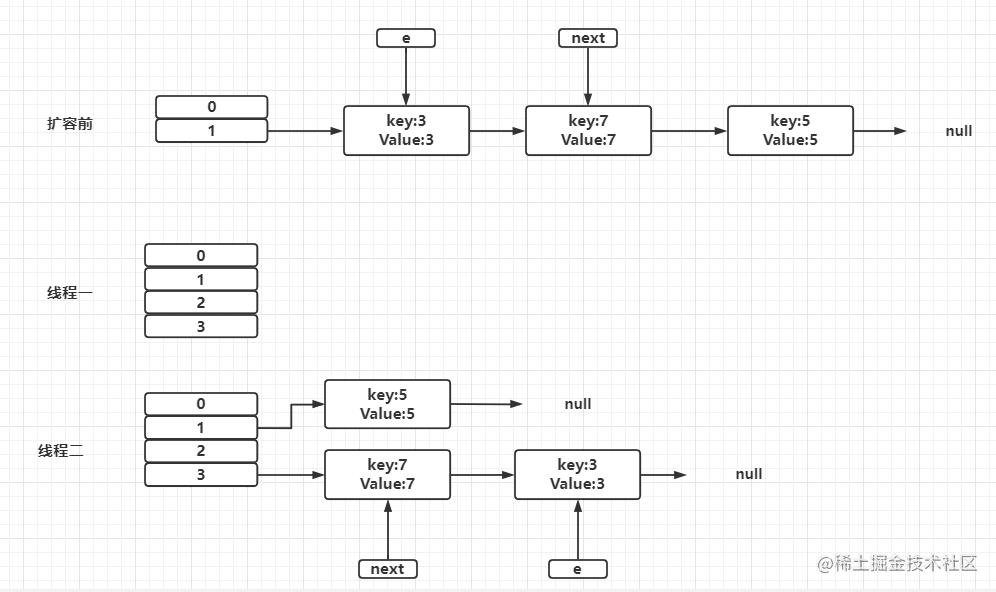从上面的图可以看到，因为线程1的 e 指向了 key(3)，而 next 指向了 key(7)，在线程 2 rehash 后，就指向了线程2 rehash 后的链表。然后线程1被唤醒了：

1. 执行e.next = newTable[i]，于是 key(3)的 next 指向了线程1的新 Hash 表，因 为新 Hash 表为空，所以e.next = null，
2. 执行newTable[i] = e，所以线程1的新 Hash 表第一个元素指向了线程2新 Hash 表的 key(3)。好了，e 处理完毕。
3. 执行e = next，将 e 指向 next，所以新的 e 是 key(7)

1. 现在的 e 节点是 key(7)，首先执行Entry next = e.next,那么 next 就是 key(3)了
2. 执行e.next = newTable[i]，于是key(7) 的 next 就成了 key(3)
3. 执行newTable[i] = e，那么线程1的新 Hash 表第一个元素变成了 key(7) 4. 执行e = next，将 e 指向 next，所以新的 e 是 key(3)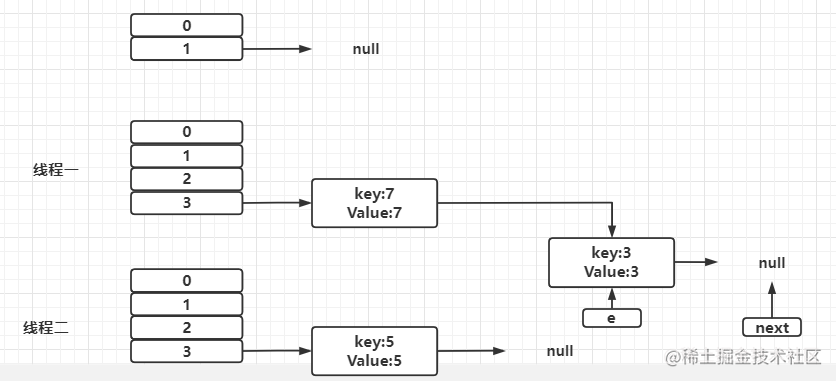1. 现在的 e 节点是 key(3)，首先执行Entry next = e.next,那么 next 就是 null
2. 执行e.next = newTable[i]，于是key(3) 的 next 就成了 key(7)
3. 执行newTable[i] = e，那么线程1的新 Hash 表第一个元素变成了 key(3)
4. 执行e = next，将 e 指向 next，所以新的 e 是 key(7)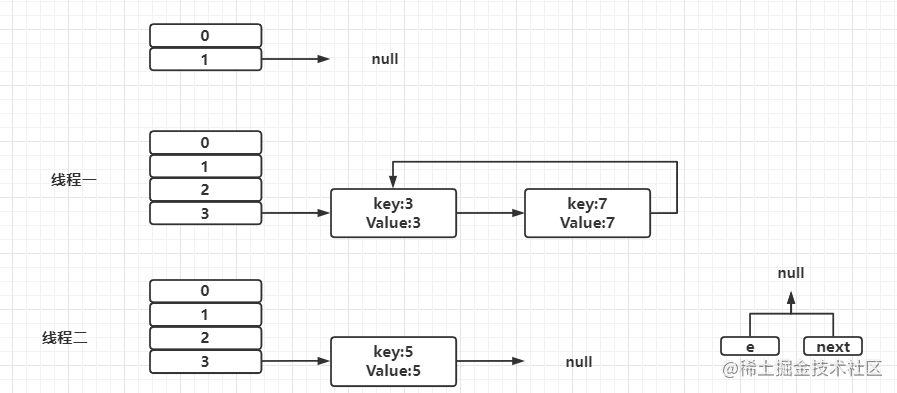#### JDK1.8中的HashMap

##### 重要成员属性
``````static final int DEFAULT_INITIAL_CAPACITY = 1 << 4; // aka 16

static final int MAXIMUM_CAPACITY = 1 << 30;

static final float DEFAULT_LOAD_FACTOR = 0.75f;
// 链表转红黑树的阈值
static final int TREEIFY_THRESHOLD = 8;
// 红黑树转链表的阈值
static final int UNTREEIFY_THRESHOLD = 6;
// 链表转红黑树时hash表最小容量阈值，达不到优先扩容
static final int MIN_TREEIFY_CAPACITY = 64;

``````(exp(-0.5) * pow(0.5, k) / factorial(k)).

0:    0.60653066
1:    0.30326533
2:    0.07581633
3:    0.01263606
4:    0.00157952
5:    0.00015795
6:    0.00001316
7:    0.00000094
8:    0.00000006
more: less than 1 in ten million

##### put()方法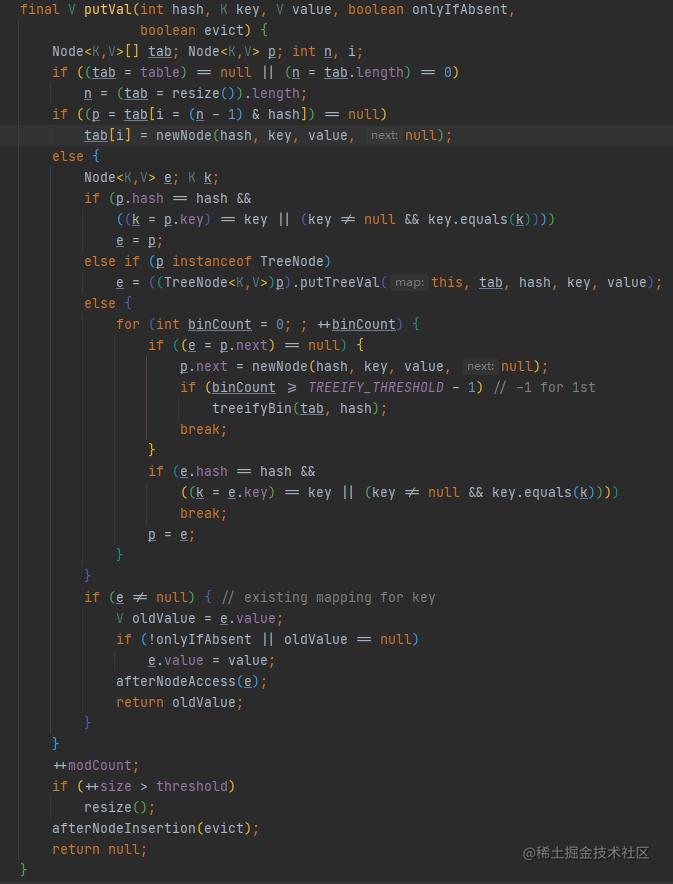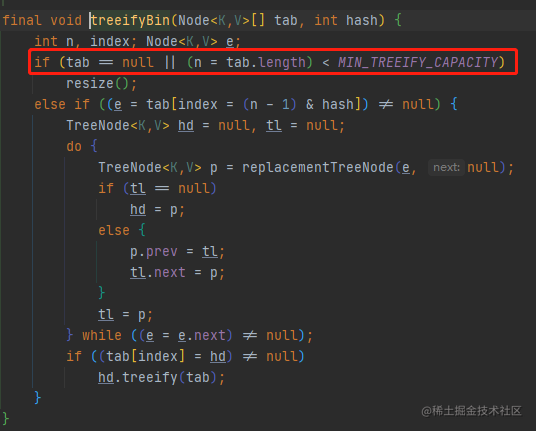``````final Node<K,V>[] resize() {
Node<K,V>[] oldTab = table;
int oldCap = (oldTab == null) ? 0 : oldTab.length;
int oldThr = threshold;
int newCap, newThr = 0;
if (oldCap > 0) {
if (oldCap >= MAXIMUM_CAPACITY) {
threshold = Integer.MAX_VALUE;
return oldTab;
}
else if ((newCap = oldCap << 1) < MAXIMUM_CAPACITY &&
oldCap >= DEFAULT_INITIAL_CAPACITY)
newThr = oldThr << 1; // double threshold
}
else if (oldThr > 0) // initial capacity was placed in threshold
newCap = oldThr;
else {               // zero initial threshold signifies using defaults
newCap = DEFAULT_INITIAL_CAPACITY;
}
if (newThr == 0) {
float ft = (float)newCap * loadFactor;
newThr = (newCap < MAXIMUM_CAPACITY && ft < (float)MAXIMUM_CAPACITY ?
(int)ft : Integer.MAX_VALUE);
}
threshold = newThr;
@SuppressWarnings({"rawtypes","unchecked"})
Node<K,V>[] newTab = (Node<K,V>[])new Node[newCap];
table = newTab;
if (oldTab != null) {
for (int j = 0; j < oldCap; ++j) {
Node<K,V> e;
if ((e = oldTab[j]) != null) {
oldTab[j] = null;
if (e.next == null)
newTab[e.hash & (newCap - 1)] = e;
else if (e instanceof TreeNode)
((TreeNode<K,V>)e).split(this, newTab, j, oldCap);
else { // preserve order
Node<K,V> loHead = null, loTail = null;
Node<K,V> hiHead = null, hiTail = null;
Node<K,V> next;
do {
next = e.next;
if ((e.hash & oldCap) == 0) { //用低位指针
if (loTail == null)
else
loTail.next = e;
loTail = e;
}
else {                     // 高位指针
if (hiTail == null)
else
hiTail.next = e;
hiTail = e;
}
} while ((e = next) != null);
if (loTail != null) {
loTail.next = null;
}
if (hiTail != null) {
hiTail.next = null;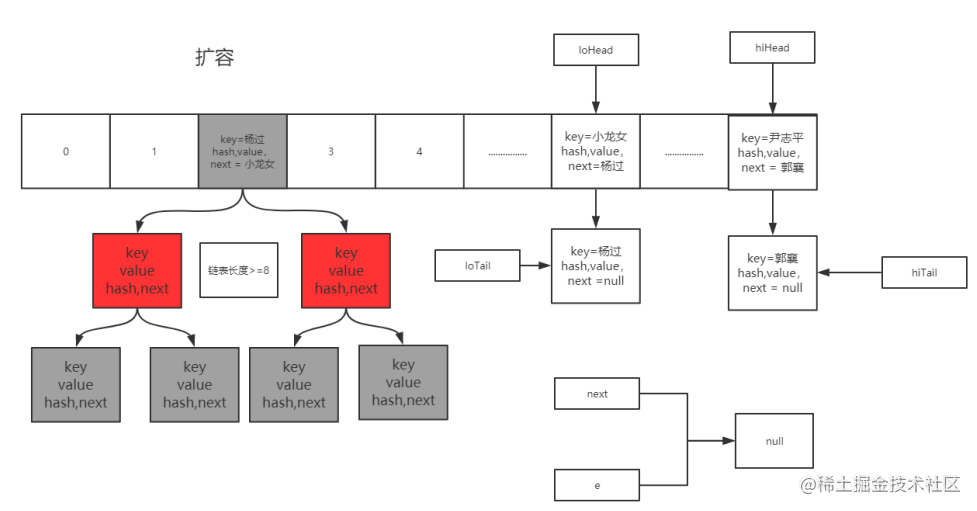jdk8中put方法过程图：